Quality and Process Methods > Process Capability > Statistical Details for the Process Capability Platform > Capability Indices for Nonnormal Distributions: Percentile and Z-Score Methods
Publication date: 08/13/2020

## Capability Indices for Nonnormal Distributions: Percentile and Z-Score Methods

This section describes how capability indices are calculated for nonnormal distributions. Two methods are described: the Percentile (also known as ISO/Quantile) method and the Z-Score (also known as Bothe/Z-scores) method. When you select a distribution for a nonnormal process variable, you can fit a parametric distribution or a nonparametric distribution. You can use either the Percentile or the Z-Score methods to calculate capability indices for the process variable of interest. However, unless you have a very large amount of data, a nonparametric fit might not accurately reflect behavior in the tails of the distribution.

Note: For both the Percentile and the Z-Score methods, if the data are normally distributed, the capability formulas reduce to the formulas for normality-based capability indices.

The descriptions of the two methods use the following notation:

LSL = Lower specification limit

USL = Upper specification limit

T = Target value

### Percentile (ISO/Quantile) Method

The percentile method replaces the mean in the standard capability formulas with the median of the fitted distribution and the 6σ range of values with the corresponding percentile range. The method is described in AIAG (2005).

Denote the α*100th percentile of the fitted distribution by Pα. Then Percentile method capability indices are defined as follows: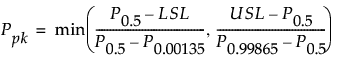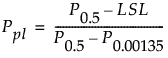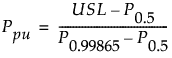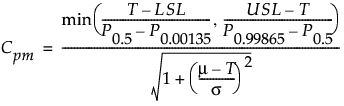### Z-Score (Bothe/Z-Scores) Method

The Z-Score method transforms the specification limits to values that have the same probabilities on a standard normal scale. It computes capability measures that correspond to a normal distribution with the same risk levels as the fitted nonnormal distribution.

Let F denote the fitted distribution for a process variable with lower and upper specification limits given by LSL and USL. Define equivalent standard normal specification limits as follows: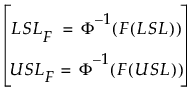Then the Z-Score method capability indices are defined as follows: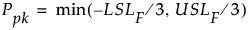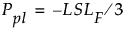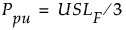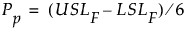Note: Because Cpm is a target-based measure, it cannot be calculated using the Z-Scores method.

Note: For very capable data, F(LSL) or F(USL) can be so close to zero or one, respectively, that LSLF or USLF cannot be computed. In these cases, JMP automatically switches from the Z-Score method to the Percentile method by default. This gives more meaningful capability indices. To turn off this default setting, select File > Preferences > Platforms > Process Capability.